### Home > PC > Chapter 8 > Lesson 8.2.3 > Problem8-112

8-112.
1. The circle below has a radius of 5 cm. The figure inscribed in the circle is a rectangle with width 2x. Express each of the following in terms of x. Homework Help ✎

2.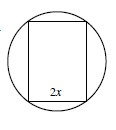1. The perimeter of the rectangle.

2. The area of the rectangle.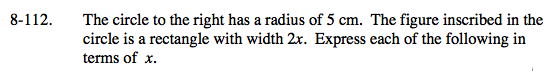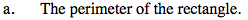Draw a diagonal. If the radius is 5, how long is the diagonal?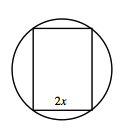Use the Pythagorean Theorem to find the length L.

$(2x)^2+L^2=10^2$

$L^2=100-4x^2$

$L=\sqrt{100-4x^2}$

$L=\sqrt{4(25-x^2)}$

$L=2\sqrt{25-x^2}$

Perimeter = 2(width) + 2(length)

$\text{Perimeter}=2(2x)+2(2\sqrt{25-x^2})$

$\text{Perimeter}=4x+4\sqrt{25-x^2}$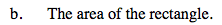Area = (Length)(Width)

$\text{Area}=(2x)(2\sqrt{25-x^2})$

$\text{Area}=4x\sqrt{25-x^2}$